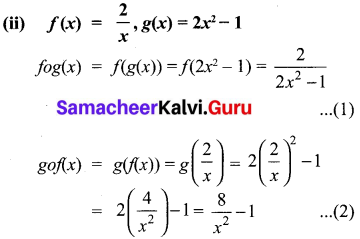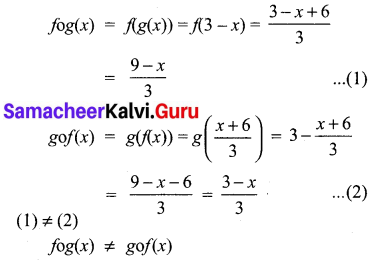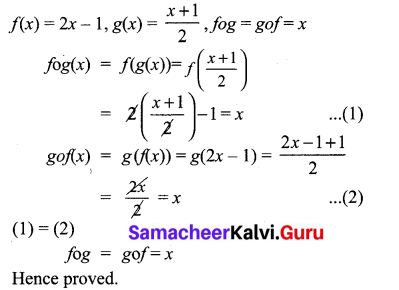## Tamilnadu Samacheer Kalvi 10th Maths Solutions Chapter 1 Relations and Functions Ex 1.5

Exercise 1.5 Class 10 Maths Samacheer Question 1
Using the functions f and g given below, find fog and gof. Check whether fog = gof.
(i) f(x) = x – 6, g(x) = x2
(ii) f(x) = $$\frac{2}{x}$$, g(x) = 2x2 – 1
(iii) f(x) = $$\frac{x+6}{3}$$ g(x) = 3 – x
(iv) f(x) = 3 + x, g(x) = x – 4
(v) f(x) = 4x2– 1,g(x) = 1 + x
Solution:
(i) f(x) = x – 6, g(x) = x2
fog(x) = f(g(x)) = f(x2) = x2 – 6 …………….. (1)
gof(x) = g(f(x)) = g(x – 6) = (x – 6)2
= x2 + 36 – 12x = x2 – 12x + 36 ……………… (2)
(1) ≠ (2)
∴ fog(x) ≠ gof(x)(iii) f(x) = $$\frac{x+6}{3}$$ g(x) = 3 – x(iv) f(x) = 3 + x, g(x) = x – 4
fog(x) = f(g(x)) = f(x – 4) = 3 + x – 4
= x – 1 ………… (1)
gof(x) = g(f(x)) = g(3 + x) = 3 + x – 4
= x – 1 ……………… (2)
Here fog(x) = gof(x)

(v) f(x) = 4x2 – 1, g(x) = 1 + x
fog(x) = f(g(x)) = f(1 + x) = 4(1 + x)2 – 1
= 4(1 + x2 + 2x) – 1 = 4 + 4x2 + 8x – 1
= 4x2 + 8x + 3 ……………. (1)
gof(x) = g(f(x)) = g(4x2 – 1)
= 1 + 4x2 – 1 = 4x2 …………….. (2)
(1) ≠ (2)
∴ fog(x) ≠ gof(x)

10th Maths Exercise 1.5 Samacheer Kalvi Question 2.
Find the value of k, such that f o g = g o f

(i) f(x) = 3x + 2, g(x) = 6x – k
f(x) = 3x + 2 ;g(x) = 6x – k
fog = f[g(x)]
= f (6x – k)
= 3(6x – k) + 2
= 18x – 3K + 2
g0f= g [f(x)]
= g (3x + 2)
= 6(3x + 2) – k
= 18x + 12 – k
But given fog = gof.
18x – 3x + 2 = 18x + 12 – k
-3k + 2 = 12 – k
-3 k + k = 12-2
-2k = 10
k = $$\frac { -10 }{ 2 }$$ = -5
The value of k = -5

(ii) f(x) = 2x – k, g(x) = 4x + 5
f(x) = 2x – k ; g(x) = 4x + 5
fog = f[g(x)]
= f(4x + 5)
= 2(4x + 5) – k
= 8x + 10 – k
gof = g [f(x)]
= g(2x – k)
= 4(2x – k) + 5
= 8x – 4k + 5
But fog = gof
8x + 10 – k = 8x – 4k + 5
-k + 4k = 5 – 10
3k = -5
k = $$\frac { -5 }{ 3 }$$
The value of k = $$\frac { -5 }{ 3 }$$

10th Maths Exercise 1.5 Answers Question 3.
if f(x) = 2x – 1, g(x) = $$\frac{x+1}{2}$$, show that fog = gof = x
Solution:
f(x) = 2x – 1, g(x) = $$\frac{x+1}{2}$$, fog = gof = xEx 1.5 Class 10 Samacheer Question 4.
(i) If f (x) = x2 – 1, g(x) = x – 2 find a, if g o f(a) = 1.
(a) Find k, if f(k) = 2k -1 and
fof (k) = 5.
(i) f(x) = x2 – 1 ; g(x) = x – 2 .
gof = g [f(x)]
= g(x2 – 1)
= x2 – 1 – 2
= x2 – 3
given gof (a) = 1
a2 – 3 = 1 [But go f(x) = x2 – 3]
a2 = 4
a = $$\sqrt { 4 }$$ = ± 2
The value of a = ± 2

(ii) f(k) = 2k – 1 ; fof(k) = 5
fof = f[f(k)]
= f(2k – 1)
= 2(2k – 1) – 1
= 4k – 2 – 1
= 4k – 3
fof (k) = 5
4k – 3 = 5
4k = 5 + 3
4k = 8
k = $$\frac { 8 }{ 4 }$$ = 2
The value of k = 2

10th Maths Exercise 1.5 Question 5.
Let A,B,C ⊂ N and a function f: A → B be defined by f(x) = 2x + 1 and g : B → C be defined by g(x) = x2. Find the range of fog and gof
Solution:
f(x) = 2x + 1
g(x) = x2
fog(x) = fg(x)) = f(x2) = 2x2 + 1
gof(x) = g(f(x)) = g(2x + 1) = (2x + 1)2
= 4x2 + 4x + 1
Range of fog is
{y/y = 2x2 + 1, x ∈ N}
Range of gof is
{y/y = (2x + 1)2, x ∈ N}.

10th Maths 1.5 Exercise Question 6.
Let f(x) = x2 – 1. Find (i) fof (ii) fofof
f(x) = x2 – 1
(i) fof = f[f{x)]
= f(x2 – 1)
= (x2 – 1)2 – 1
= x4 – 2x2 + 1 – 1
= x4 – 2x2

(ii) fofof = fof[f(x)]
= fof (x2 – 1)
= f(x2 – 1)2 – 1
= f(x4 – 2x2 + 1 – 1)
= f (x4 – 2x2)
fofof = (x4 – 2x2)2 – 1

10th Maths Exercise 1.5 In Tamil Question 7.
If f: R → R and g : R → R are defined by f(x) = x5 and g(x) = x4 then check if f,g are one-one and fog is one-one?
Solution:
f(x) = x5
g(x) = x4
fog = fog(x) = f(g(x)) = f(x4)
= (x4)5 = x20
f is one-one, g is not one-one.
∵ g(1) = 14 = 1
g(-1) = ( -1)4 = 1
Different elements have same images
fog is not one-one. [∵ fog (1) = fog (-1) = 1]

Exercise 1.5 Class 10 Maths Question 8.
Consider the functions f(x), g(x), h(x) as given below. Show that
(f o g) o h = f o(g o h) in each case.
(i) f(x) = x – 1, g(x) = 3x + 1 and h(x) = x2
(ii) f(x) = x2, g(x) = 2x and h(x) = x + 4
(iii) f(x) = x – 4, g(x) = x2 and h(x) = 3x – 5
(i) f(x) = x – 1, g (x) = 3x + 1, h(x) = x2
fog (x) = f[g(x)]
= f(3x + 1)
= 3x + 1 – 1
fog = 3x
(fog) o h(x) = fog [h(x)] ,
= fog (x2)
= 3(x2)
(fog) oh = 3x2 …..(1)
goh (x) = g[h(x)]
= g(x2)
= 3(x2) + 1
= 3x2 +1
fo(goh) x = f [goh(x)]
= f[3x2 + 1]
= 3x2 + 1 – 1
= 3x2 ….(2)
From (1) and (2) we get
(fog) oh = fo (goh)
Hence it is verified

(ii) f(x) = x2 ; g (x) = 2x and h(x) = x + 4
(fog) x = f[g(x)]
= f (2x)
= (2x)2
= 4x2
(fog) oh (x) = fog [h(x)]
= fog (x + 4)
= 4(x + 4)2
= 4[x2 + 8x + 16]
= 4x2 + 32x + 64 …. (1)
goh (x) = g[h(x)]
= g(x + 4)
= 2(x + 4)
= 2x + 8
fo(goh) x = fo [goh(x)]
= f[2x + 8]
= (2x + 8)2
= 4×2 + 32x + 64 …. (2)
From (1) and (2) we get
(fog) oh = fo(goh)

(iii) f(x) = x – 4 ; g (x) = x2; h(x) = 3x – 5
fog (x) = f[g(x)]
= f(x2)
= x2 – 4
(fog) oh (x) = fog [h(x)]
= fog (3x – 5)
= (3x – 5)2 – 4
= 9x2 – 30x + 25 – 4
= 9x2 – 30x + 21 ….(1)
goh (x) = g[h(x)]
= g(3x – 5)
= (3x – 5)2
= 9x2 + 25 – 30x
fo(goh)x = f[goh(x)]
= f[9x2 – 30x + 25]
= 9x2 – 30x + 25 – 4
= 9x2 – 30x + 21 ….(2)
From (1) and (2) we get
(fog) oh = fo(goh)

Samacheer Kalvi 10th Maths Book Solutions Question 9.
Let f ={(-1, 3),(0, -1),(2, -9)} be a linear function from Z into Z . Find f(x).
Solution:
f ={(-1, 3), (0, -1), 2, -9)
f(x) = (ax) + b ………… (1)
is the equation of all linear functions.
∴ f(-1) = 3
f(0) = -1
f(2) = -9
f(x) = ax + b
f(-1) = -a + b = 3 …………… (2)
f(0) = b = -1
-a – 1 = 3 [∵ substituting b = – 1 in (2)]
-a = 4
a = -4
The linear function is -4x – 1. [From (1)]

Samacheer Kalvi 10th Maths Exercise 1.5 Question 10.
In electrical circuit theory, a circuit C(t) is called a linear circuit if it satisfies the superposition principle given by C(at1 + bt2) = aC(t1) + bC(t2), where a, b are constants. Show that the circuit C(t) = 31 is linear.